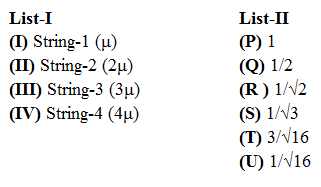### JEE (Advanced) 2019 Paper-2 Question 15

Instructions

A musical instrument is made using four different metal strings, 1, 2, 3 and 4 with mass per unit length $$\mu, 2\mu, 3\mu$$ and $$4\mu$$ respectively. The instrument is played by vibrating the strings by varying the free length in between the range $$L_0$$ and $$2L_0$$. It is found that in string-1 $$(\mu)$$ at free length $$L_0$$ and tension $$T_0$$ the fundamental mode frequency is $$f_0$$.
List-I gives the above four strings while list-II lists the magnitude of some quantity.Question 15